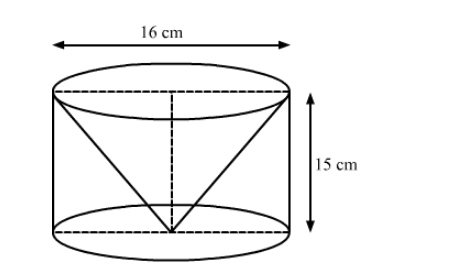# From a solid cylinder whose height is 15 cm and diameter 16 cm, a conical cavity of the same height and same diameter is hollowed out.

Question:

From a solid cylinder whose height is 15 cm and diameter 16 cm, a conical cavity of the same height and same diameter is hollowed out. Find the total surface area of the remaining solid. (Use π">π = 3.14)

Solution:We have,

Height of the cylinder $=$ Height of the cone $=h=15 \mathrm{~cm}$ and

Radius of the cylinder $=$ Radius of the cone $=r=\frac{16}{2}=8 \mathrm{~cm}$

Also, the slant height of the cone, $l=\sqrt{h^{2}+r^{2}}$

$=\sqrt{15^{2}+8^{2}}$

$=\sqrt{225+64}$

$=\sqrt{289}$

$=17 \mathrm{~cm}$

Now,

The total surface area of the remaining solid = CSA of the cone $+$ CSA of the cylinder $+$ Area of the base

$=\pi r l+2 \pi r h+\pi r^{2}$

$=\pi r(l+2 h+r)$

$=3.14 \times 8 \times(17+2 \times 15+8)$

$=3.14 \times 8 \times 55$

$=1381.6 \mathrm{~cm}^{2}$

So, the total surface area of the remaining solid is 1381.6 cm2.

Disclaimer: The answer given in the textbook is incorrect. The same has been corrected above.